### What are Hilbert and Banach spaces?

It is dangerous to live without knowing what Hilbert and Banach spaces are. One might be spontaneously quizzed on one’s recollection, and it would be very embarrassing to not know the answer! Thankfully, we need only remember that Hilbert spaces are the objects which allow us to generalize linear algebra and apply it to analytic settings.

The basic object of linear algebra is a vector space. How might we take vector spaces and “add in” elements of analysis? Notice that we while we can add and subtract vectors together, we do not yet have a sense of continuity in this vector space.

Therefore, to bring in this missing concept, simply require that the vector space is complete with respect to convergent sequences. Note that the notion of convergence of Cauchy sequences inherently requires the notion of a norm. For Hilbert spaces, we require that the norm is actually induced by an inner product so that we retain some notion of “angular distance.” (Hilbert spaces are very useful in physics, where the inner product of two complex wavefunctions gives some notion of a ‘transition probability’ from one to the other, vaguely analogous to some sense of angular distance.)

That is it for Hilbert spaces!

To obtain Banach spaces, we simply relax the requirement that the norm is induced by an inner product and instead allow arbitrary norms (the space must still be complete with respect to convergent sequences, though). A natural set of examples is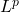spaces, which are only Hilbert for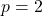, but Banach for all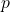.

To differentiate the two, one need only remember the differences between inner products and norms. Inner products are functions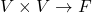which induce a natural norm and satisfy various reasonable properties whereas norms, being somewhat more general, are just functions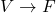which satisfy various other reasonable properties. As one might imagine, the presence of an inner product, which allows you to relate pairs of vectors in angular-distance-like ways, is more restrictive and adds more structure to the underlying vector space than does a norm, which just assigns a number to each vector.

The structure of Hilbert spaces is quite useful; for example, closed convex subsets of Hilbert spaces have a unique element that minimize the (induced) norm, whereas in Banach spaces there is no guarantee as to either existence or uniqueness of such an element. Similarly, in Hilbert spaces, closed vector subspaces have orthogonal complements… and so on and so forth.

February 10th, 2023 | Posted in Math

### One Response to “What are Hilbert and Banach spaces?”

1.Jackson Jules Says:

Every Hilbert space is a Banach space, but not every Banach space is a Hilbert space. Stackexchange has a short thread on Banach spaces that are not Hilbert spaces (https://math.stackexchange.com/questions/692522/a-banach-space-that-is-not-a-hilbert-space#:~:text=Banach%20spaces%20that%20are%20not,element%20of%20the%20space%20itself.)